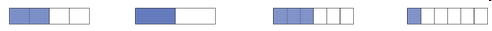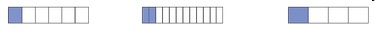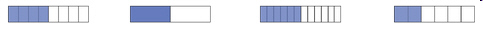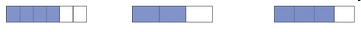3.NF.3.B.-Test1B Recognize and generate simple equivalent fractions
 Name:    3.NF.3.B.-Test1B Recognize and generate simple equivalent fractions

Multiple Choice
Identify the choice that best completes the statement or answers the question.

1.

Which of the following fraction bars would not be equivalent to the others?a. 3/6 c. 2/4 b. 1/6 d. 1/2

2.

Which fraction is  equivalent to 7/21
 a. 3/7 c. 1/3 b. 1/7 d. 2/7

3.

Which fraction is not equivalent to 1/4?
 a. 4/16 c. 3/12 b. 2/3 d. 2/8

4.

Which fraction is not equivalent to 1/6?
 a. 2/12 c. 4/24 b. 3/18 d. 4/5

5.

Which fraction would be equivalent to 2/3?
 a. 4/6 c. 1/4 b. 2/9 d. 1/3

6.

Which fraction would be equivalent to 1/2?
 a. 1/3 c. 2/4 b. 1/8 d. 3/4

7.

Which of the following fractions are not equivalent to each other?a. 1/6 c. 2/12 b. 1/4 d. all

8.

Which of the following fractions are not equivalent to the others?a. 4/8 c. 1/2 b. 6/12 d. 2/6

9.

Which of the following fraction bars would not be equivalent to the others?a. 2/4 c. 2/3 b. 4/6 d. all

10.

Which fraction is not equivalent to 1/2?
 a. 2/4 c. 4/8 b. 2/5 d. 3/6